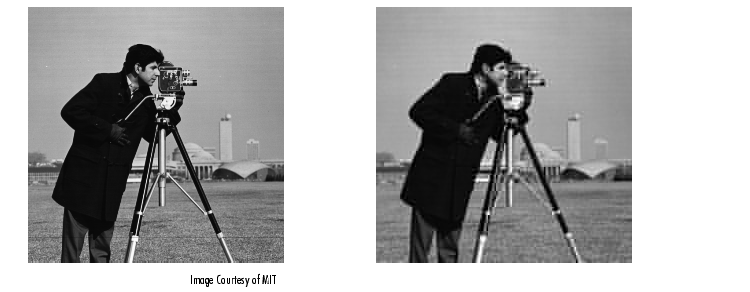Image Processing Toolbox User's GuideDCT and Image Compression

In the JPEG image compression algorithm, the input image is divided into 8-by-8 or 16-by-16 blocks, and the two-dimensional DCT is computed for each block. The DCT coefficients are then quantized, coded, and transmitted. The JPEG receiver (or JPEG file reader) decodes the quantized DCT coefficients, computes the inverse two-dimensional DCT of each block, and then puts the blocks back together into a single image. For typical images, many of the DCT coefficients have values close to zero; these coefficients can be discarded without seriously affecting the quality of the reconstructed image.

The example code below computes the two-dimensional DCT of 8-by-8 blocks in the input image, discards (sets to zero) all but 10 of the 64 DCT coefficients in each block, and then reconstructs the image using the two-dimensional inverse DCT of each block. The transform matrix computation method is used.

• ```I = imread('cameraman.tif');
I = im2double(I);
T = dctmtx(8);
B = blkproc(I,[8 8],'P1*x*P2',T,T');
mask = [1   1   1   1   0   0   0   0
1   1   1   0   0   0   0   0
1   1   0   0   0   0   0   0
1   0   0   0   0   0   0   0
0   0   0   0   0   0   0   0
0   0   0   0   0   0   0   0
0   0   0   0   0   0   0   0
0   0   0   0   0   0   0   0];Although there is some loss of quality in the reconstructed image, it is clearly recognizable, even though almost 85% of the DCT coefficients were discarded. To experiment with discarding more or fewer coefficients, and to apply this technique to other images, try running the demo function `dctdemo`.The DCT Transform Matrix Radon Transform